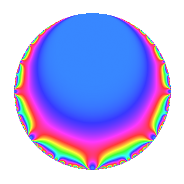# Properties

 Label 44.1.dLevel 44 Weight 1 Character orbit d Rep. character $$\chi_{44}(21,\cdot)$$ Character field $$\Q$$ Dimension 1 Newforms 1 Sturm bound 6 Trace bound 0

# Related objects

## Defining parameters

 Level: $$N$$ = $$44 = 2^{2} \cdot 11$$ Weight: $$k$$ = $$1$$ Character orbit: $$[\chi]$$ = 44.d (of order $$2$$ and degree $$1$$) Character conductor: $$\operatorname{cond}(\chi)$$ = $$11$$ Character field: $$\Q$$ Newforms: $$1$$ Sturm bound: $$6$$ Trace bound: $$0$$

## Dimensions

The following table gives the dimensions of various subspaces of $$M_{1}(44, [\chi])$$.

Total New Old
Modular forms 4 1 3
Cusp forms 1 1 0
Eisenstein series 3 0 3

The following table gives the dimensions of subspaces with specified projective image type.

$$D_n$$ $$A_4$$ $$S_4$$ $$A_5$$
Dimension 1 0 0 0

## Trace form

 $$q$$ $$\mathstrut -\mathstrut q^{3}$$ $$\mathstrut -\mathstrut q^{5}$$ $$\mathstrut +\mathstrut O(q^{10})$$ $$q$$ $$\mathstrut -\mathstrut q^{3}$$ $$\mathstrut -\mathstrut q^{5}$$ $$\mathstrut +\mathstrut q^{11}$$ $$\mathstrut +\mathstrut q^{15}$$ $$\mathstrut -\mathstrut q^{23}$$ $$\mathstrut +\mathstrut q^{27}$$ $$\mathstrut -\mathstrut q^{31}$$ $$\mathstrut -\mathstrut q^{33}$$ $$\mathstrut -\mathstrut q^{37}$$ $$\mathstrut +\mathstrut 2q^{47}$$ $$\mathstrut +\mathstrut q^{49}$$ $$\mathstrut +\mathstrut 2q^{53}$$ $$\mathstrut -\mathstrut q^{55}$$ $$\mathstrut -\mathstrut q^{59}$$ $$\mathstrut -\mathstrut q^{67}$$ $$\mathstrut +\mathstrut q^{69}$$ $$\mathstrut -\mathstrut q^{71}$$ $$\mathstrut -\mathstrut q^{81}$$ $$\mathstrut -\mathstrut q^{89}$$ $$\mathstrut +\mathstrut q^{93}$$ $$\mathstrut -\mathstrut q^{97}$$ $$\mathstrut +\mathstrut O(q^{100})$$

## Decomposition of $$S_{1}^{\mathrm{new}}(44, [\chi])$$ into irreducible Hecke orbits

Label Dim. $$A$$ Field Image CM RM Traces $q$-expansion
$$a_2$$ $$a_3$$ $$a_5$$ $$a_7$$
44.1.d.a $$1$$ $$0.022$$ $$\Q$$ $$D_{3}$$ $$\Q(\sqrt{-11})$$ None $$0$$ $$-1$$ $$-1$$ $$0$$ $$q-q^{3}-q^{5}+q^{11}+q^{15}-q^{23}+q^{27}+\cdots$$